Suppose we want to make a game to improve the ability of user to memorize only four very difficult Japanese Kanji characters. For simplicity, we call this Kanji character a, b, c, and d and in our example, user makes only 10 trials. In this example, we will use Reward and Punishment learning histogramto make the program can learn adaptively to user response.

In the first table, user input is recorded as Right if the answer is correct and Wrong if the user make mistake. In our example, the first generated character is a and the user give wrong answer. The second generated character is b and the user answer correctly. The fourth generated character is a again and this time our user pass correct answer, and so on. The user can only pass one input for every trial. Thus in each column of the table, only single entry with binary values is allowed, either Right or Wrong.

Table 1 User response

 trial no 0 1 2 3 4 5 6 7 8 9 10 a Wrong Right Wrong Right b Right c Wrong Right Right d Wrong Right

Let us define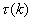to cumulatively counts the number entry generated by the program, for each character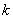. The trick here is to make the non-zero number of entry because we will use this number for denominator of our probability distribution. To do that, we set the entry for all character as 1 at trial 0. Table 2 shows the cumulative count. At trial 1, the program generated question for character a, thus the number of entry of a is added by 1, while the other character remain. At trial 2, the generated character is b, thus, the number of entry fro character b is added by one, while the number of entry for other characters remain the same. Repeat the process for all trials.

Table 2 Cumulative number of entry for each character,trial no 0 1 2 3 4 5 6 7 8 9 10 a 1 2 2 2 3 3 3 3 3 4 5 b 1 1 2 2 2 2 2 2 2 2 2 c 1 1 1 2 2 2 2 3 4 4 4 d 1 1 1 1 1 2 3 3 3 3 3

Histogram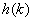counts the number of occurrence that user answer charactercorrectly or wrong, divided by the total number of entry. For our simple game, let us define arbitrary weight 1 for correct answers and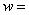0.1 for wrong answer. Initially, the histogram contain only zero occurrence, or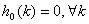. The histogram is formulated as(A)

In the table 3, we show the histogram of weighted answer. It simply accumulates the user response of table 1. The bottom of the table gives the summation of each column. Initially, at trial number zero, the histogram is empty to characterize no bias. Notice that only dummy trial number 0 produces zero sums and no real trial will have zero sums.

Table 3 Histogram of reward and punishment,trial no 0 1 2 3 4 5 6 7 8 9 10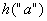0 0.1 0.1 0.1 1.1 1.1 1.1 1.1 1.1 1.2 2.2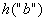0 0 1 1 1 1 1 1 1 1 1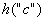0 0 0 0.1 0.1 0.1 0.1 1.1 2.1 2.1 2.1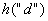0 0 0 0 0 0.1 1.1 1.1 1.1 1.1 1.1 Sum 0 0.1 1.1 1.2 2.2 2.3 3.3 4.3 5.3 5.4 6.4

If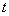is the current total number of trials (i.e. can be answered right and wrong), the probability that characterhas been successfully answered is(A)

This probability of success is calculated by dividing each entry of Table 3 by the entry of table 2. The sum of each column is the denominator of equation (A) while each entry of the new table is the nominator of equation (A). The result of computation is shown in Table 4. The summation of probability in each trial is always 100%.

Table 4 Probability that characterhas been successfully answered,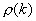trial no 0 1 2 3 4 5 6 7 8 9 10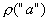100% 9% 8% 40% 38% 29% 23% 21% 18% 24%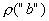0% 91% 83% 55% 52% 39% 31% 28% 30% 27%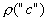0% 0% 8% 5% 5% 4% 23% 30% 31% 29%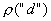0% 0% 0% 0% 5% 29% 23% 21% 22% 20%

We need to divide the histogramby the cumulatively entry numberto normalize the result. Without the division to the cumulatively entry number, we may get incorrect response. For example, a character, which has record of one right answer and one wrong answer, may get lower failure probability than a character with only one right answer. This response is reversed what is expected and is happen because the total number of entry of the two characters are not the same. To fix this mistake, we normalize the probability by the total number of entry.

Our target in this game is to get the cumulative probability of failure. We need to design the program response such that the characters that have small probability of correct answers will be asked more frequently. In other words, we want to get the failure distribution instead of success distribution. The probability distribution we have so far represents the success answers.

The probability distribution of failure is given by normalizing one minus the probability of success. Table 5 gives probability distribution of failure of user response based on equation (B).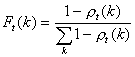(B)

Table 5 Probability distribution of failure

 Trial no 1 2 3 4 5 6 7 8 9 10 F(a) 0% 30% 31% 20% 21% 24% 26% 26% 27% 25% F(b) 33% 3% 6% 15% 16% 20% 23% 24% 23% 24% F(c) 33% 33% 31% 32% 32% 32% 26% 23% 23% 24% F(d) 33% 33% 33% 33% 32% 24% 26% 26% 26% 27%

To examine the adaptive learning, we shall compare user response in table 1 and the Probability distribution of failure (represents the computer response) in Table 5. The first trial is unstable to be compared because it has only single data. In the second trial, character b get correct answer and the probability of failure reduce so much it has smaller chance to be asked again. In trial 3, the user give wrong answer to character c, and the probability of failure between character a and c is now the same (because both has the same record of one wrong answer) while character d has higher probability. This computer response is very good because it will give better chance to the character that has not been generated to be shown. Trial 4 gives correct answer to character a make probability of a is lower (i.e. less chance to be shown). Notice that in trial 4 the character a has 1 wrong and 1 right answer, while character b only has one right answer. The failure probability of b is smaller than a. This is the exact response what we want. If we do not normalize the probability of success by the cumulatively entry number(as in Equation (A)), the response of the computer will be incorrect. Trial 5 shows character d in which the user gives wrong answer. The probability of c and d is now the same because they have the same record one wrong answer. The rest of the trials goes with similar story as above.

.Table 6 Cumulative Probability of failure

 trial no 1 2 3 4 5 6 7 8 9 10 Ca 0% 30% 31% 20% 21% 24% 26% 26% 27% 25% Cb 33% 33% 36% 35% 37% 44% 49% 50% 51% 50% Cc 67% 67% 67% 67% 68% 76% 74% 74% 74% 73% Cd 100% 100% 100% 100% 100% 100% 100% 100% 100% 100%

Table 6 shows the cumulative probability of failure from Table 5. The inverse of the cumulative probability distribution of failure can be used for Monte Carlo algorithm as in thesimple game without learning. For example, in trial 10, suppose the random number generated by computer is denoted by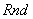, then

Show character a if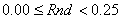Show character b if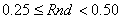Show character c if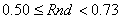Show character d if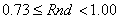Preferable reference for this tutorial is

Teknomo, Kardi (2015) Learning Algorithm Tutorials. https:\\people.revoledu.com\kardi\ tutorial\Learning\